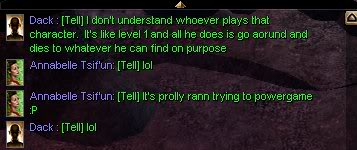Anphillia ExodusNWN 1 Faction roleplay PW using CEP v2.3HomeFAQSearchMemberlistUsergroupsRegisterLog in

 Anphillia Exodus :: Changes and Fixes :: Classes :: Bard Share

# Bard Song..AuthorMessage

StaffJoin date : 2009-04-29
Location : UKSubject: Bard Song..Wed Apr 29, 2009 3:59 am Bard Song has been altered on this mod. Here is how it works now:Quote :Class Level = 40Perform Rank = 100Attack = 5Damage = 6Will = 5Fortitude = 5Reflex = 5HP = 60AC = 7Skill Bonus = 20Quote :Class Level = 39Perform Rank = 95Attack = 5Damage = 5Will = 5Fortitude = 5Reflex = 5HP = 50AC = 6Skill Bonus = 18Quote :Class Level = 34Perform Rank = 90Attack = 4Damage = 5Will = 4Fortitude = 5Reflex = 4HP = 45AC = 6Skill Bonus = 17Quote :Class Level = 30Perform Rank = 90Attack = 4Damage = 4Will = 4Fortitude = 4Reflex = 4HP = 40AC = 6Skill Bonus = 16Quote :Class Level = 29Perform Rank = 90Attack = 3Damage = 3Will = 3Fortitude = 3Reflex = 3HP = 38AC = 4Skill Bonus = 16Quote :Class Level = 28Perform Rank = 85Attack = 3Damage = 3Will = 3Fortitude = 2Reflex = 2HP = 38AC = 4Skill Bonus = 14Quote :Class Level = 27Perform Rank = 85Attack = 3Damage = 3Will = 3Fortitude = 2Reflex = 2HP = 36AC = 4Skill Bonus = 14Quote :Class Level = 26Perform Rank = 80Attack = 3Damage = 3Will = 3Fortitude = 2Reflex = 2HP = 36AC = 4Skill Bonus = 14Quote :Class Level = 25Perform Rank = 75Attack = 3Damage = 3Will = 3Fortitude = 2Reflex = 2HP = 34AC = 4Skill Bonus = 13Quote :Class Level = 24Perform Rank = 70Attack = 3Damage = 3Will = 3Fortitude = 2Reflex = 2HP = 32AC = 4Skill Bonus = 10Quote :Class Level = 23Perform Rank = 65Attack = 2Damage = 3Will = 3Fortitude = 2Reflex = 2HP = 30AC = 4Skill Bonus = 10Quote :Class Level = 22Perform Rank = 60Attack = 2Damage = 3Will = 3Fortitude = 2Reflex = 2HP = 32AC = 5Skill Bonus = 11Quote :Class Level = 21Perform Rank = 55Attack = 2Damage = 3Will = 3Fortitude = 2Reflex = 2HP = 30AC = 5Skill Bonus = 9Quote :Class Level = 20Perform Rank = 50Attack = 2Damage = 3Will = 3Fortitude = 2Reflex = 2HP = 28AC = 5Skill Bonus = 8Quote :Class Level = 19Perform Rank = 45Attack = 2Damage = 3Will = 3Fortitude = 2Reflex = 2HP = 26AC = 5Skill Bonus = 7Quote :Class Level = 18Perform Rank = 40Attack = 2Damage = 3Will = 3Fortitude = 2Reflex = 2HP = 24AC = 5Skill Bonus = 6Quote :Class Level = 17Perform Rank = 35Attack = 2Damage = 3Will = 3Fortitude = 2Reflex = 2HP = 22AC = 5Skill Bonus = 5Quote :Class Level = 16Perform Rank = 30Attack = 2Damage = 3Will = 3Fortitude = 2Reflex = 2HP = 20AC = 5Skill Bonus = 4Quote :Class Level = 15Perform Rank = 24Attack = 2Damage = 3Will = 2Fortitude = 2Reflex = 2HP = 16AC = 4Skill Bonus = 3Quote :Class Level = 14Perform Rank = 21Attack = 2Damage = 3Will = 1Fortitude = 1Reflex = 1HP = 16AC = 3Skill Bonus = 2Quote :Class Level = 11Perform Rank = 18Attack = 2Damage = 2Will = 1Fortitude = 1Reflex = 1HP = 8AC = 2Skill Bonus = 2Quote :Class Level = 8Perform Rank = 15Attack = 2Damage = 2Will = 1Fortitude = 1Reflex = 1HP = 8AC = 0Skill Bonus = 1Quote :Class Level = 6Perform Rank = 12Attack = 1Damage = 2Will = 1Fortitude = 1Reflex = 1HP = 0AC = 0Skill Bonus = 1Quote :Class Level = 3Perform Rank = 9Attack = 1Damage = 2Will = 1Fortitude = 1Reflex = 0HP = 0AC = 0Skill Bonus = 0Quote :Class Level = 2Perform Rank = 6Attack = 1Damage = 1Will = 1Fortitude = 0Reflex = 0HP = 0AC = 0Skill Bonus = 0Quote :Class Level = 1Perform Rank = 3Attack = 1Damage = 1Will = 0Fortitude = 0Reflex = 0HP = 0AC = 0Skill Bonus = 0_________________Anphillia Exodus; Gameworld Page - Vote nicely!Bard Song..Page 1 of 1
 Similar topics» Kentucky Rain - The Rooster Song - Video!
» Bolton Squad's Christmas Song
» Ducks unveil their baseball uniforms
» Song to stir the team and fans
» love this song.

Permissions in this forum:You cannot reply to topics in this forumJump to: Select a forum||--Announcements|--Downloads|--Anphillia OOC Forum|   |--Server Rules|   |--Bug Reports|   |--Feedback|   |--General Discussion|   |--Off Topic|   |--Credits|   |--Anphillia IC Forum|   |--Character Stories|   |--World Events|   |--Fort Cleaven|   |--Fort Axfell|   |--Changes and Fixes    |--Classes    |   |--Arcane archer    |   |--Assassin    |   |--Barbarian    |   |--Bard    |   |--Blackguard    |   |--Champion of Torm    |   |--Cleric    |   |--Druid    |   |--Dwarven defender    |   |--Fighter    |   |--Harper scout    |   |--Monk    |   |--Paladin    |   |--Pale master    |   |--Purple dragon knight    |   |--Ranger    |   |--Rogue    |   |--Shadowdancer    |   |--Shifter    |   |--Sorcerer/Wizard    |   |--Weapon Master    |   |--Red dragon disciple    |       |--General Abilities    |--Spells    |--Subraces    |--Associates